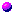Analysis WebNotes
arrow_back arrow_forward

# Class Contents

### What is Analysis and Why Study It?

Analysis is one of the large divisions of modern mathematics. (Algebra, applied mathematics, topology, and discrete mathematics are some others.)

Analysis is the study of limits. Anything in mathematics which has limits in it uses ideas of analysis. Some of the examples which will be important in this course are sequences, infinite series, derivatives of functions, and integrals.

As you know from calculus, limits are the basis of understanding integration and differentiation, and, as you also know from calculus, these things are the basis of everything in the world you could ever need to know.

Probably, when you took calculus, you spent a lot of time learning techniques of how to integrate and differentiate, and actually learning about limits was dealt with in a fairly short time. (see note) But limits are a very rich, and very subtle concept. So, if you like, you can think of a theme in this course as "lift up the hood of calculus and take a look at what's inside". But you'll also find a lot of ideas that are completely new, and that are tied in with thinking about what we mean by a limit.

The idea of taking limits is very old, and just as old are some of the problems which limits can raise. The ancient Greeks calculated the area of a circle using a limiting argument. But they also knew of Zeno's Paradox , which uses a suble misunderstanding of limits to "prove" that all motion is impossible. The modern study of analysis grew out of a dissatisfaction with the early intellectual basis of calculus.

When calculus was first discovered, it enabled a very thorough understanding of many different physical phenomena, from the motion of the planets to a bouncing ball. What was unsatisfactory wasn't the results it produced, but the mathematical arguments that calculus was based on. These appealed to ideas of infinitesimals , which mathematicians found impossible to tie down to a clear meaning.

Because the idea of limits was notorious for leading to paradoxes (like Zeno's Paradox ), or else to two different answers, with equally plausible justifications , analysis was developed to put calculus and limits on a firm footing. The heritage of analysis, for this reason, is rigor. The way you can be confident that you are on the right path, is that every little step can be checked carefully, and every assumption can be traced back to a few simple rules (axioms). You will probably find that this course puts more emphasis on rigor than any previous math courses which you've had.

### Mathematical Rigor and Axioms

Axioms are a set of rules for a mathematical theory. Everything you do in that branch of mathematics has to be able to be broken down into steps which are all applications of the axioms.

The benefits of this are that you can analyze an argument in a proof carefully, and be completely confident that it's right, by checking that every step conforms to the axioms.

Thus, the purpose of having axioms in mathematics is to enable you to be confident that your proofs are correct. The next topic highlights some of the difficulties you can get into with even quite simple facts when you don't have clear basic principles (which is what axioms are) to appeal to.

### Group Exercise: Archimedes's Property

As an illustration of the need for firm principles to base our work with real numbers on, consider the following theorem (which we will prove later on in Chapter 1).

Theorem. (The Archimedean Property of the Reals) Let x be a real number. Then there always exists a whole number n which is bigger than xIn groups of two or three, talk about the theorem, and how you would prove it. Take turns to explain why it's true, while the others try to spot what hidden assumptions you're making.

The truth of the theorem isn't in doubt for a minute! The point is that when you start to think about it, you begin to see how difficult it is to prove it to your complete satisfaction.

Question: What is it that makes it so difficult to tie down this (let's face it, obvious) fact?

The next couple of sections will deal with building up the basic rules for the real numbers, but we'll come back to the Archimedean Property, and prove it in Proposition 2.3.